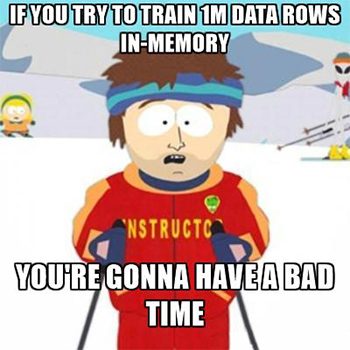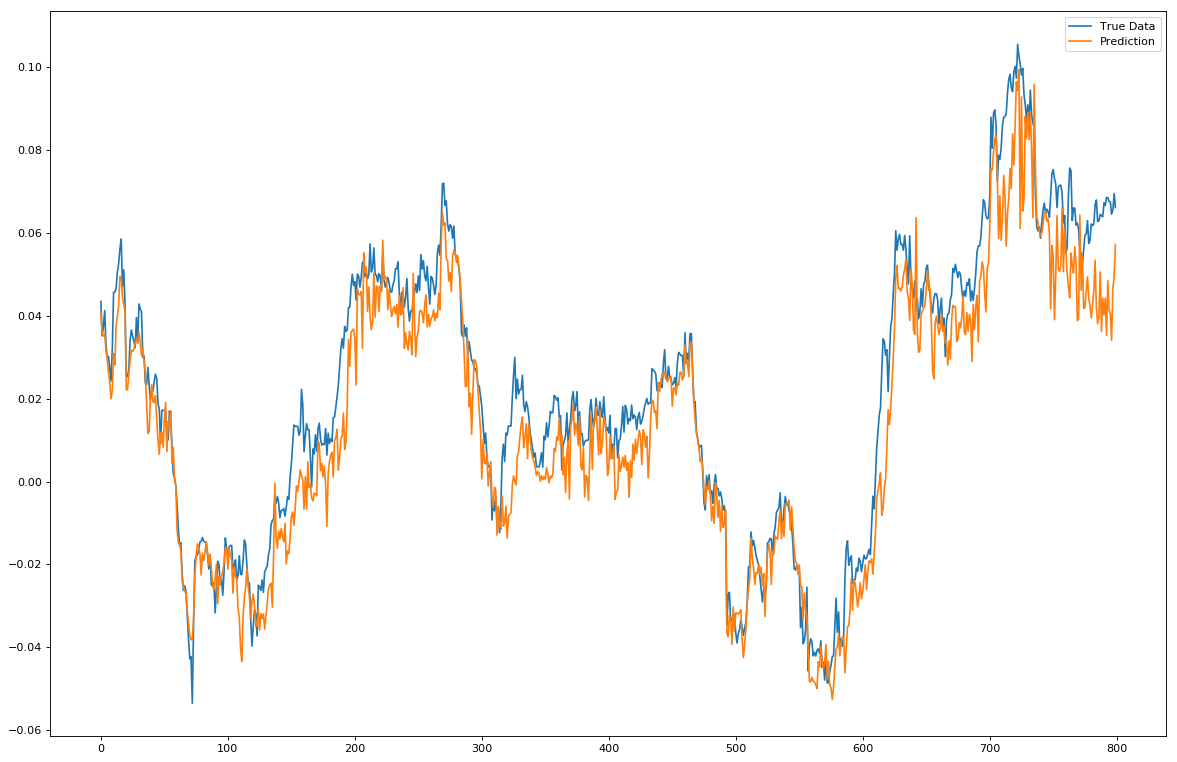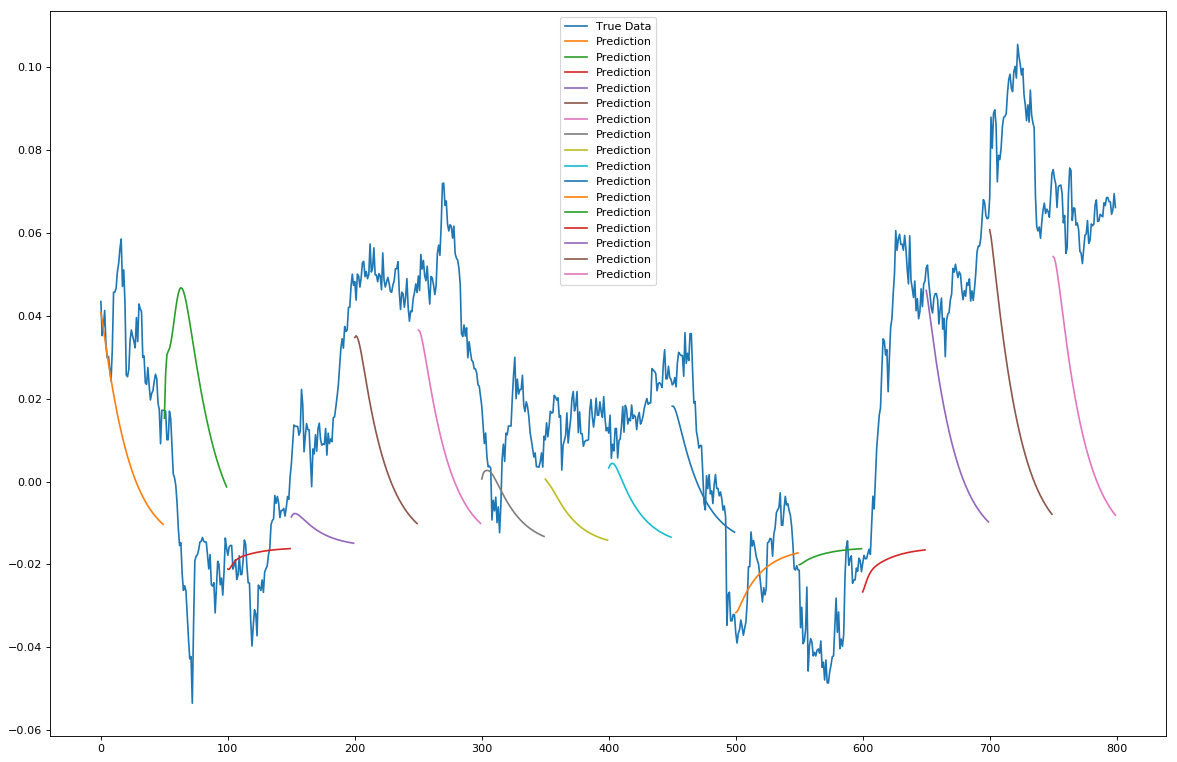# Multidimensional LSTM Networks to Predict Bitcoin Price

Sat 15th Jul 2017

This article builds on the work from my last one on LSTM Neural Network for Time Series Prediction. If you haven't read that, I would highly recommend checking it out to get to grips with the basics of LSTM neural networks from a simple non-mathematical angle.

Considering the recent re-surge in buzz around the ridiculous Bitcoin bubble Bitcoin currency, I thought I would theme this article topically around predicting the price and momentum of Bitcoin using a multidimensional LSTM neural network that doesn’t just look at the price, but also looks at the volumes traded of BTC and the currency (in this case USD) and creates a multivariate sequential machine learning model out of it.

As anyone who's been on a date with me knows; I find small talk boring, so let's just jump right into it!

## Dataset Time

The first thing we will need is the data. Luckily, Kaggle have a fun dataset of minute-by-minute historical data set from Bitcoin which includes 7 factors. Perfect!

We will however need to normalise this dataset before feeding it into our network of LSTMs. We will do this as per the previous article where we take a sliding window of size N across the data and re-base to data to be returns from 0 where .

Now this being a multidimensional approach, we are going to be doing this sliding window approach across all of our dimensions. Normally, this would be a pain in the ass. Luckily the Python Pandas library comes to the rescue! We can represent each window as a Pandas dataframe and we can then perform the normalisation operation across the whole dataframe (i.e. across all columns).

The other thing you will notice with this dataset is that especially at the beginning, the data is not very clean. There’s a lot of NaN values floating around in various columns which would not make our model particularly happy. We’ll take a lazy approach to fixing this: when we create our window we'll check if any value in the window is a NaN. If it is, we will swipe left throw away the window and move on to the next one.

Whilst we're here, let's make these functions into a self contained class called ETL (extract, transform, load) and save it as etl.py that way we can call this whole data loader as a library.

Here is the core code from our clean_data() fundtion:

``````num_rows = len(data)
x_data = []
y_data = []
i = 0
while((i+x_window_size+y_window_size) <= num_rows):
x_window_data = data[i:(i+x_window_size)]
y_window_data = data[(i+x_window_size):(i+x_window_size+y_window_size)]

#Remove any windows that contain NaN
if(x_window_data.isnull().values.any() or y_window_data.isnull().values.any()):
i += 1
continue

if(normalise):
abs_base, x_window_data = self.zero_base_standardise(x_window_data)
_, y_window_data = self.zero_base_standardise(y_window_data, abs_base=abs_base)

#Average of the desired predicter y column
y_average = np.average(y_window_data.values[:, y_col])
x_data.append(x_window_data.values)
y_data.append(y_average)
i += 1
```
```

Once we have done this, we simply make sure our LSTM model accepts sequences of shape M where M = the number of dimensions of our data, and we’re done!

Or that’s what you would think, but life is rarely ever that easy. See the first time I tried to do this, my machine shuddered to a halt and gave me a memory error. The issue, you see, comes from the fact that the Bitcoin dataset, being a minute-by-minute dataset, is quite large. When normalised it is around 1 million data windows. And loading all of these 1 million windows into Keras to train on at once makes for a pretty bad time.So how would I train on this data without adding an extra 100Gb of RAM to my machine? Furthermore, if this data grew to 100x the size adding more RAM wouldn’t exactly be feasible. Well this is where the Keras fit_generator() function comes in pretty damn handy!

Now if you don’t know anything about Python generators, STOP (hammer time) and go read up on them.

In a nutshell; a generator iterates over data of unknown (and potentially infinite) length, only passing out the next piece every time it is called. Now if you have half a brain I’m sure you can see where this would come in useful; if we can train the model one a small chunk of windows at a time, then throw away those windows once we are done with them to be replaced by the next set of windows. This trains the model with low memory utilisation. Perfect! Technically speaking, if you made the windows small enough you could even train this model on your IoT toaster machine if you really wanted to!

What we need to do then, is create a generator that creates a batch of windows to then pass to the Keras fit_generator() function. Easy, we just extend the core clean_data() code to yield (return in a generative way) batches of windows:

``````#Restrict yielding until we have enough in our batch. Then clear x, y data for next batch
if(i % batch_size == 0):
#Convert from list to 3 dimensional numpy array [windows, window_val, val_dimension]
x_np_arr = np.array(x_data)
y_np_arr = np.array(y_data)
x_data = []
y_data = []
yield (x_np_arr, y_np_arr)
```
```

## No-One Likes Re-Runs

Now the other issue I found here was the clean_data() generator that I created was taking on average 3-4 seconds to create each “batch” of windows. On its own this was acceptable as it took around 15-20mins to get through the training data batches. However, if I wanted to tweak the model and re-run it, it would take an awful long time to re-train it again.

What can we do? Well, how about pre-normalising it then saving the normalised numpy arrays of windows to a file, hopefully one that preserves the structure and is super-fast to access?

HDF5 to the rescue! Through the use of the h5py library we can easily save the clean and normalised data windows as a list of numpy arrays that takes a fraction of a second IO time to access. So let's make a function that does exactly that and call it create_clean_datafile():

``````def create_clean_datafile(self, filename_in, filename_out, batch_size=1000, x_window_size=100, y_window_size=1, y_col=0, filter_cols=None, normalise=True):
print('> Creating x & y data files...')

data_gen = self.clean_data(
filename_in,
batch_size = batch_size,
x_window_size = x_window_size,
y_window_size = y_window_size,
y_col = y_col,
filter_cols = filter_cols,
normalise = True
)

i = 0
with h5py.File(filename_out, 'w') as hf:
x1, y1 = next(data_gen)
#Initialise hdf5 x, y datasets with first chunk of data
rcount_x = x1.shape
dset_x = hf.create_dataset('x', shape=x1.shape, maxshape=(None, x1.shape, x1.shape), chunks=True)
dset_x[:] = x1
rcount_y = y1.shape
dset_y = hf.create_dataset('y', shape=y1.shape, maxshape=(None,), chunks=True)
dset_y[:] = y1

for x_batch, y_batch in data_gen:
#Append batches to x, y hdf5 datasets
print('> Creating x & y data files | Batch:', i, end='\r')
dset_x.resize(rcount_x + x_batch.shape, axis=0)
dset_x[rcount_x:] = x_batch
rcount_x += x_batch.shape
dset_y.resize(rcount_y + y_batch.shape, axis=0)
dset_y[rcount_y:] = y_batch
rcount_y += y_batch.shape
i += 1
```
```

Now we can just create a new generator function generate_clean_data() to open the hdf5 file and spit out those same normalised batches at lightning fast speed into the Keras fit_generator() function!

``````def generate_clean_data(self, filename, batch_size=1000, start_index=0):
with h5py.File(filename, 'r') as hf:
i = start_index
while True:
data_x = hf['x'][i:i+batch_size]
data_y = hf['y'][i:i+batch_size]
i += batch_size
yield (data_x, data_y)
```
```

Looking at the data however, we don’t want to add unnecessary noise with some of the dimensions. What I have done is created an argument to the create_clean_datafile() function that takes in factors (columns) to filter. With this, I’ve narrowed my data file down to a 4-dimensional time series consisting of Open, Close, Volume (BTC) and Volume (Currency). This will cut down on the time I’ll take to train the network as well. Winning!

The data is then fed into the network which has one input LSTM layer that takes in data of shape [dimensions, sequence_size, training_rows], a second LSTM layer that’s hidden, and a fully connected output layer with a tanh function for spitting out the next predicted normalised return percentage.

Training is done by calculating the steps_per_epoch based on our number of epochs and our train/test split as specified in our configs JSON file.

Testing is done in a similar way, using the same generator as for training and utilising the Keras predict_generator() function. The only extra thing we need to add in when predicting our test set is a generator function that iterates the generator and splits out the x and y outputs. This is because the Keras predict_generator() function only takes the x inputs and wouldn't know what to do with a tuple of x and y values. However we still want the y values (true data), so we store them in a separate list as we want to use them for plotting against to be able to visualize our results compared to the true data. We then do the same but rather than predict on a a step-by-step basis we initialise a window of size 50 with the first prediction, and then keep sliding the window along the new predictions taking them as true data, so we slowly start predicting on the predictions and hence are forecasting the next 50 steps forward.

Finally, we save the test set predictions and test set true y values in a HDF5 file again so we can easily access them in the future without re-running everything, should the model turn out to be useful. We then plot the results on 2 matplotlib charts. One showing the daily 1-step-ahead predictions, the other showing 50-steps ahead predictions.

## Hello Sweet Bitcoin Profit

We then go for the forecasting of Bitcon price! As per my last article, we will try and do two types of forecasts:

The first will be predicting on a point-by-point basis, that is predicting the t+1 point, then shifting the window of true data and predicting again the next point along. Repeat. Here is the results of point-by-point predictions:The second forecast type is a t+n multiple steps ahead forecast, where we populate the shifting window with predictions initialised from a window of true data and plot N steps ahead. The results of this look like:What can we see? Well, we can see that when predicting 1 step ahead it's doing a very reasonable job. Occasionally it's out, but in general it follows the true data quite well. However, the predictions do appear far more volatile than the true data. Without doing more tests it's hard to ascertain why this might be and if a model re-parameterisation would fix this.

When predicting the trend however this model starts to fall a bit on its face. The trend doesn't seem particularly accurate to model and is inconsistent at times. However! What is interesting is the size of the predicted trend line does seem to correlate with the size of the price moves (volatility).

## Conclusion

I am going to use this section to take off my AI hat and put on my investment manager hat to explain a few key truths...

The main thing one should realise is that predicting returns is a pretty futile exercise. I mean sure, it's the holy grail of forecasting to be able to predict returns, and whilst some top end hedge funds do try to do just that by finding new alpha indicators in truth it's a pretty hard thing to do due to the huge swaths of external influences that push an asset price. In real terms it's comparable to trying to predict the next step of a random walk.

However, all is not lost and our exercise isn't completely pointless. See, whilst with limited time series data, even with multiple dimensions it's hard to predict returns, what we can see, especially from the second chart, is that there is an avenue there to predicting volatility. And not just volatility, but we could also expand that to predict market environments in a way allowing us to know what type of market environment we are currently in.

Why would this be useful? Well a lot of different strategies (which I won't go into here) work well in different market environments respectively. A momentum strategy might work well in a low vol, strongly trending environment whilst an arbitrage strategy might be more successful in producing high returns in a high vol environment. We can see that by knowing our current market environment and predicting the future market environments are key to allocating the correct strategy to the market at any given time. Whilst this is more of a general investment approach for traditional markets, the same would apply to the Bitcoin market.

So as you can see, predicting longer-term Bitcoin prices is currently (as with all types of stock markets) pretty hard and nobody can claim to do so from just the technical time-series data because there are a lot more factors that go into the price changes. Another issue which is worth touching on with the use of LSTM neural networks across a dataset like this is the fact that we are taking the whole time series data set as a stationary time series. That is to say, the properties of the time series are assumed unchanged throughout time. This is unfortunately not true as the factors that influence price changes also vary over time, so assuming a property/pattern that the network finds in the past remains true for the present day is a naive approach that doesn't necessarily hold.

There is work that can be done help with this non-stationarity issue, the leading edge research of this currently focuses on using Bayesian methods alongside of LSTMs to overcome the issue of time series non-stationarity.

But that's far out of scope for this short article. I may make another post in the future detailing the implementation of this. Check back soon!

In the meantime, feel free to browse the full code for this project on my GitHub page: Multidimensional-LSTM-BitCoin-Time-Series